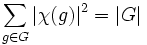# Irreducible character of degree greater than one takes value zero on some conjugacy class

## Statement

Suppose$G$ is a finite group and$\chi$ is the character of an irreducible linear representation of$G$ over$\mathbb{C}$, such that the degree of the representation (and hence, of$\chi$) is greater than one. Then, there exists an element$g \in G$ (and hence, a Conjugacy class (?)) such that$\chi(g) = 0$.

## Examples

Representation Degree Conjugacy class(es) where the character is zero Group Linear representation theory information
standard representation of symmetric group:S3 2$(1,2)$ -- class of 2-transpositions symmetric group:S3 linear representation theory of symmetric group:S3
faithful irreducible representation of dihedral group:D8 2 all the conjugacy classes of elements outside the center. dihedral group:D8 linear representation theory of dihedral group:D8
faithful irreducible representation of quaternion group 2 all the conjugacy classes of elements outside the center. quaternion group linear representation theory of quaternion group
standard representation of symmetric group:S4 3$(1,2,3)$ -- class of 3-cycles symmetric group:S4 linear representation theory of symmetric group:S4

## Facts used

1. Character orthogonality theorem
2. Sufficiently large implies splitting: In particular, this exhibits a splitting field of characteristic zero that is a finite cyclotomic extension of the rationals.
3. Cauchy-Schwartz inequality
4. Characters are algebraic integers

## Proof

Given: A finite group$G$,$\chi$ the character of an irreducible representation of degree greater than$1$ of the group$G$.$e$ is the identity element,$d = \chi(e)$ is the degree, so$d > 1$.

To prove: There exists$g \in G \setminus \{ e \}$ such that$\chi(g) = 0$.

Proof:

Step no. Assertion/construction Facts used Given data used Previous steps used Explanation
1$\sum_{g \in G} |\chi(g)|^2 = |G|$ Fact (1) Use Fact (1), and move the$1/|G|$ to the other side.
2$d^2 + \sum_{g \in G \setminus \{ e \}} |\chi(g)|^2 = |G|$ Step (1) Pull out the term for the identity element.
3$\sum_{g \in G \setminus \{ e \}} |\chi(g)|^2 = |G| - d^2 < |G| - 1$$d > 1$, i.e., the representation has degree more than one Step (2) Rearrange Step (2), use$d > 1$.
4 Suppose$K$ is a finite degree cyclotomic extension of$\mathbb{Q}$ that is a splitting field for$G$. Let$H$ be the Galois group of the field extension$K/\mathbb{Q}$. Then,$H$ acts on the set of irreducible representations, with an automorphism$\sigma$ acting by:$\varphi \mapsto (g \mapsto \sigma(\varphi(g))$
Fact (2) Fact (2) guarantees the existence of such a$K$.
5$\sum_{g \in G \setminus \{ e \}} \left[\prod_{\sigma \in H} |\sigma(\chi(g))| \right]^2 \le \prod_{\sigma \in H} \left[ \sum_{g \in G \setminus \{ e \}} |\sigma(\chi(g))|^2 \right]^{1/|H|}$ Fact (3) Steps (3), (4)
6$\sum_{g \in G \setminus \{ e \}} |N_K(\chi(g))|^2 \le \prod_{\sigma \in H} \left[ \sum_{g \in G \setminus \{ e \}} |\sigma(\chi(g))|^2 \right]^{1/|H|}$ Step (5) [SHOW MORE]
7$\sum_{g \in G \setminus \{ e \}} |\sigma(\chi(g))|^2 = |G| - d^2 < |G| - 1$ for every$\sigma \in H$ Steps (2), (4) [SHOW MORE]
8$\prod_{\sigma \in H} \left[ \sum_{g \in G \setminus \{ e \}} |\sigma(\chi(g))|^2 \right]^{1/|H|} < |G| - 1$ Step (7) [SHOW MORE]
9$\sum_{g \in G \setminus \{ e \}} |N_K(\chi(g))|^2 < |G| - 1$ Steps (6), (8) Step-combination direct
10 Each$N_K(\chi(g))$ is an integer, so each$|N_K(\chi(g))|^2$ is a nonnegative integer. Fact (4) Step (4) (definition of$K$) [SHOW MORE]
11 For some$g \in G \setminus \{ e \}$,$N_K(\chi(g)) = 0$ Steps (9), (10) [SHOW MORE]
12 For some$g \in G \setminus \{ e \}$,$\chi(g) = 0$ Step (11) Follows from Step (11) and the observation that$N_K(x) = 0 \iff x = 0$.